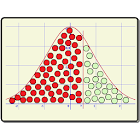Normal Distribution

All Android applications categories

All Android games categories# Normal Distribution

by: 10 8

8 Users
rating

## Screenshots

Description

It calculates probabilities using the normal distribution, the standard one with mean 0 and standard deviation 1, as any other one that you wan to introduce, with mean and standard deviation personalized.

It is included the possibility of calculating:
* Probability of which a quantity is over the Normal one.
* Probability of which a quantity is below the Normal one.
* Probability of which the variable is understood between two quantities.

This app is considered as a useful tool for people who want to do calculations of probabilities inside a Normal distribution, it is much more quickly than the classic table.

from 10 reviews

"Great"

8